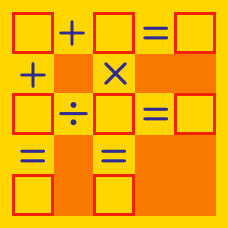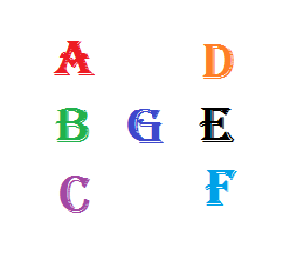Logic

Arithmetic Puzzles: Level 2 Challenges

$\large 1 \, \square \, 2 \, \square \, 3 \, \square \, 4 = 10$

There are $4^3 = 64$ ways in which we can fill the squares with $+ , - , \times , \div$.

How many ways would make the equation true?

Note:
You are not allowed to use parenthesis.
Obey the order of operations.

$\left(\color{#20A900}{\square}5\right)^2 = \color{#3D99F6}{\square}25$

Each square above represents a positive integer. Let $m$ and $n$ denote the values that fill in the green and blue squares, respectively, satisfying the equation. Then what is the relationship between $m$ and $n?$

Details and Assumptions:

• This is an arithmetic puzzle, where $1 \square$ would represent the 2-digit number 19 if $\square = 9$. It does not represent the algebraic expression $1 \times \square$.In the figure above, each letter represents a distinct single digit among $0, 1, 2, \ldots, 9.$ They are positioned in such a way that $\large A\times B\times C=B\times G\times E=D\times E\times F.$

What digit does the letter $G$ represent?

$\large \square + \square\square+\square\square\square+\square\square\square\square$

Place each of the digits 0 through 9, without repetition, in the boxes above. Then what is the maximum possible sum?

Details and Assumptions:

• This is an arithmetic puzzle, where $1 \square$ would represent the 2-digit number 19 if $\square = 9$. It does not represent the algebraic expression $1 \times \square$.

$\LARGE \square^{\square^{\square^{\square}}}$

You are given that the numbers $1,2,3,4$ are to be filled in the square boxes as shown above (without repetition), forming an exponent towers? . Over all $4!=24$ possible arrangements, let $S$ be the minimal value that is achieved. How many arrangements of these numbers would produce the value of S?

Details and Assumptions

As an explicit example, a possible value of the resultant number is $\large 2^{3^{1^4}} = 8$.

×